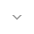belongs to Maven artifact com.android.support:support-compat:28.0.0-alpha1

# PathInterpolatorCompat

``` public final class PathInterpolatorCompat ```
``` extends Object ``` ``` ```

 java.lang.Object ↳ android.support.v4.view.animation.PathInterpolatorCompat

Helper for creating path-based `Interpolator` instances. On API 21 or newer, the platform implementation will be used and on older platforms a compatible alternative implementation will be used.

## Summary

### Public methods

``` static Interpolator``` ``` create(Path path) ```

Create an `Interpolator` for an arbitrary `Path`.

``` static Interpolator``` ``` create(float controlX1, float controlY1, float controlX2, float controlY2) ```

Create an `Interpolator` for a cubic Bezier curve.

``` static Interpolator``` ``` create(float controlX, float controlY) ```

Create an `Interpolator` for a quadratic Bezier curve.

### Inherited methodsFrom class ``` java.lang.Object ```

## Public methods

### create

`Interpolator create (Path path)`

Create an `Interpolator` for an arbitrary `Path`. The `Path` must begin at `(0, 0)` and end at `(1, 1)`. The x-coordinate along the `Path` is the input value and the output is the y coordinate of the line at that point. This means that the Path must conform to a function `y = f(x)`.

The `Path` must not have gaps in the x direction and must not loop back on itself such that there can be two points sharing the same x coordinate.

Parameters
`path` `Path`: the `Path` to use to make the line representing the `Interpolator`

Returns
`Interpolator` the `Interpolator` representing the `Path`

### create

```Interpolator create (float controlX1,
float controlY1,
float controlX2,
float controlY2)```

Create an `Interpolator` for a cubic Bezier curve. The end points `(0, 0)` and `(1, 1)` are assumed.

Parameters
`controlX1` `float`: the x coordinate of the first control point of the cubic Bezier

`controlY1` `float`: the y coordinate of the first control point of the cubic Bezier

`controlX2` `float`: the x coordinate of the second control point of the cubic Bezier

`controlY2` `float`: the y coordinate of the second control point of the cubic Bezier

Returns
`Interpolator` the `Interpolator` representing the cubic Bezier curve

### create

```Interpolator create (float controlX,
float controlY)```

Create an `Interpolator` for a quadratic Bezier curve. The end points `(0, 0)` and `(1, 1)` are assumed.

Parameters
`controlX` `float`: the x coordinate of the quadratic Bezier control point

`controlY` `float`: the y coordinate of the quadratic Bezier control point

Returns
`Interpolator` the `Interpolator` representing the quadratic Bezier curve

[]
[]